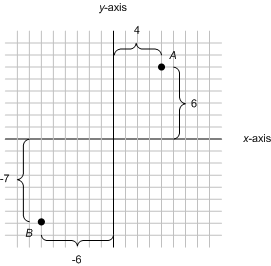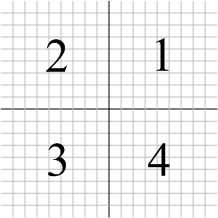Classrooms Curricula References Textbooks Site Map

# Graphing in Two DimensionsGraphing in two dimensions is a simple extension of the graphing that you do in one dimension, i.e. on a number line. To work in two dimensions, we'll need two number lines that cross at right angles. So we can tell them apart, we'll call the horizontal one the x-axis and the vertical one the y-axis. Now we can specify the location of a point, called its coordinates, by counting the number of units that we have to go over and the number of units we have to go up to get to the point.

If you look at the graph to the right, the coordinates of point A, are (4, 6) because you have to go four units over and 6 units up to get to it. Negative values work exactly the same in two dimensions as they did on a number line. Point B is located at coordinates (-6, -7) because you have to go six units to the left and 7 units down to get to it.Notice that the two axes create four equal sections. To make the four parts, called quadrants, easier to talk about, each one is given a number like the ones in the diagram on the right.